## || Civil Engineer Must Know ||

Hello Guys , welcome to engineering brother civil engineering website so today we will discuss All classification of aggregates used in actual construction of civil engineering structures.

Here are all the type discuss below...

## 1)Classification of Aggregates based on weight

Base on weight aggregate are classified as follow

A)Normal Weight Aggregate

B)Light Weight Aggregate

C)Heavy Weight Aggregate

lets briefly discuss all type

### A) Normal Weight Aggregate

This aggregate is used in all types of general concrete, with specific densities ranging from 2.5 to 2.7, and producing concrete with a density of 2300 kg / m³ to 2600 kg / m³.

Normal weight aggregate contain following two type..

### 1) Natural Aggregate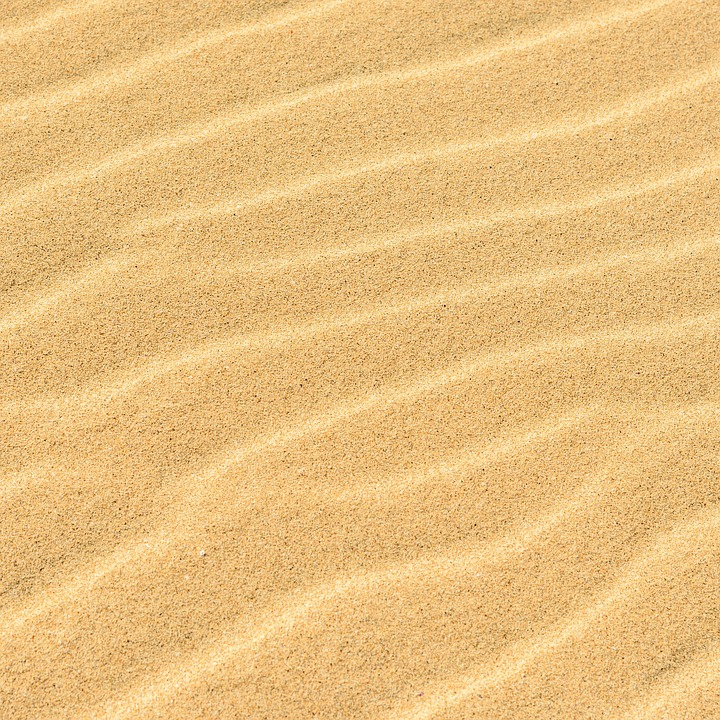SandGravelgranite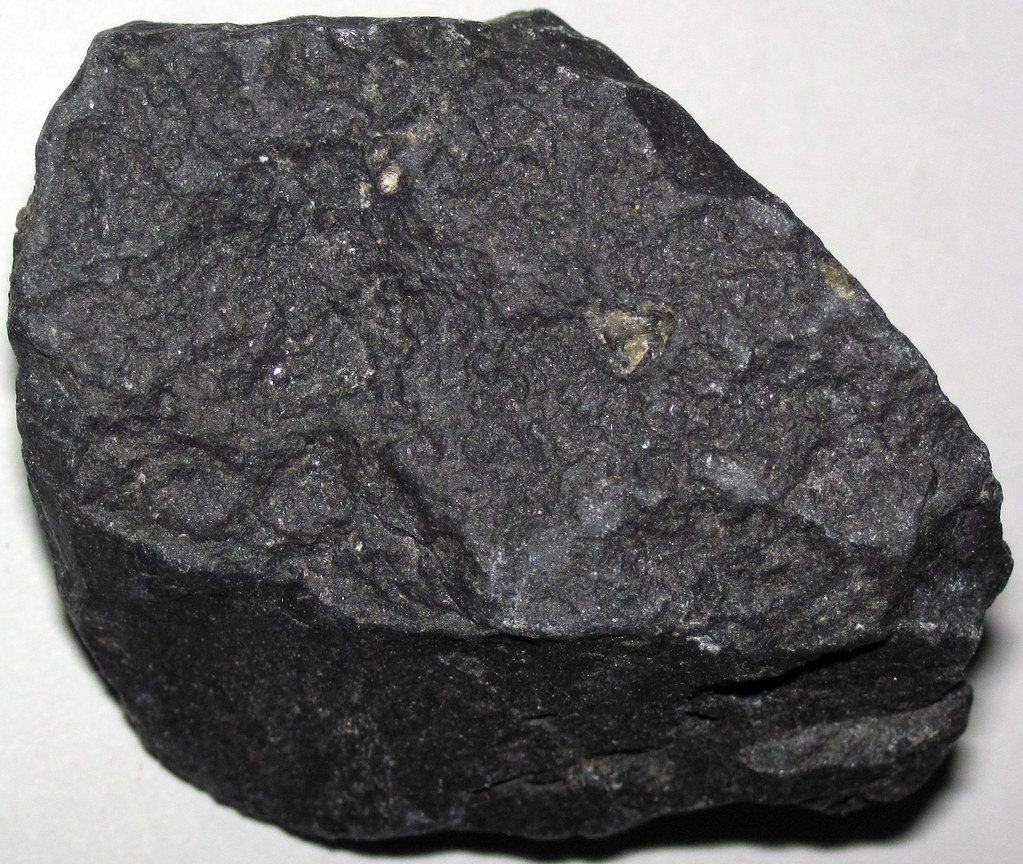basaltQuartzite

### 2)Artificial Aggregate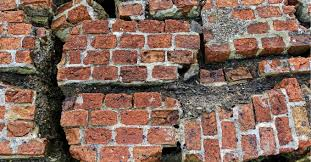Broken BrickSlag

### B) Light Weight Aggregate

The bulk density of this aggregate is 1200 kg / m³.   The weight of concrete decreases as the aggregate is lighter.  Such concrete has properties like thermal insulation and fire resistance.

Light weight aggregate contain following two type..

### 1) Natural Aggregate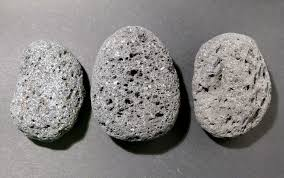PumicescoriaSawdust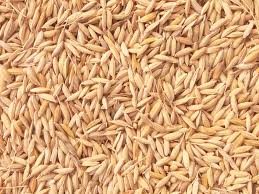rice husk

### 2) Artificial Aggregatefoamed slagClayexpanded shale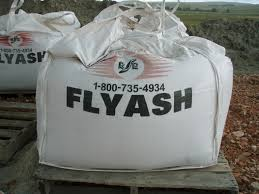FlyAsh

### C) Heavy Weight Aggregate

The density of concrete made from such aggregate is about 5000 kg / m³, which is used to protect the nuclear industry (nuclear reactor, X-ray, gamma-ray construction etc.) from radiation.  The aggregate of iron absorbs neutrons.BariteHematiteMagnetite

## 2)Classification of Aggregates Based on Size

base on Size aggregate are classified as following

A) Course  Aggregate
B)Fine aggregate
C) Single Size Aggregate:
D) Plum Concrete:

A) Course  Aggregate

size of aggregate more than  4.75 mm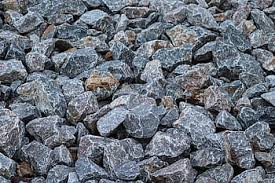course aggregate

B)Fine aggregate

size of aggregate less than and equal to 4.75 mmsand

*In concrete, the size of the largest gravel should be chosen as far as possible so that minimum cement is used and the workability of the concrete is improved.

*Less use of cement leads to less heat of hydration and less stress due to expansion and contraction in concrete.

*While Using a larger size of kappachi reduces the surface area so that the ratio decreases and the strength increases.

Difference between Course and Fine Aggregate:

Fine Aggregate

(i) Size <4.75 mm
(ii) Bulking increases.  (Up to 40%)
(iii) The function of is to fill the cavities between the CA
(iv) Sand, crushed gravel, crushed rock, gravel

Course Aggregate

(i) Size> 4.75 mm
(ii) Bulking decreases which is not taken into account.
(iii) The function of CA is to reinforce concrete
(iv) Crushed gravel, gravel etc.

C) Single Size Aggregate:

A single size aggregate in an aggregate when most of the quantities are of the same size is called a single size aggregate.  Particles of many other sizes are absent in such aggregates.

E.g.  A single size aggregate of 20 mm means that most of the aggregate passes through the 20 mm IS sieve and most of the volume stays on the 10 mm IS sieve.

D) Plum Concrete:

Stones of 150 mm or more size can be used in plain concrete.  Such concrete is called 'plum concrete'.  Such stones are called "plum".  Stones up to 20% of the total size of the concrete can be used.  Concrete vibration is done by placing stones at a certain distance on a concrete bed of about 60 cm thickness.  So that the stones sink into the concrete.plum

This method was used in the construction of Koyna Dam in Maharashtra.

## 3) Classification of Aggregates Based on Shape (IS: 383 - 1970):

Aggregates can be divided into five shapes

(i) Rounded
(ii) Irregular - Partially rounded
(iii) Angular
(iv) Leaf - Flaky
(v) Elongated

(i)Rounded

Since the surface area of ​​a spherical aggregate is less, less cement is required, gives more workability less grip between two aggregates.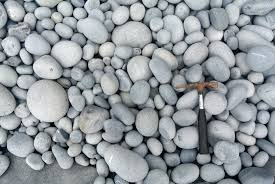Rounded Aggregate

The void ratio ranges from 32 to 33%.

E.g.  , River or beach gravel, desert and beach sand

(ii) Irregular - Partly rounded:

Grip between such aggregates is good.  The void ratio is 35 to 38%.
Eg.  Pit Sands and gravels.Irregular Aggregate

(iii)Angular:

The angles of such aggregates are sharp.  The void ratio is 38 to 40% so more cement is used.
Good grip between aggregates increases the strength of concrete.Angular aggregate

(iv) Flaky:

When the thickness of kapchi is less than its average size (60%) it is called flaky.
6) Elongated Aggregates

When the length of the kapchi is more than 9/5 (1.8 times) of its average size, it is elongated.  Both flaky and long aggregates reduce the versatility of concrete.  The durability of concrete decreases.  The proportion of flaky and long aggregates in concrete should not exceed 15%.

## 4)Classification of Aggregates Based on Texture  (IS: 382 - 1970):

aggregates can be divided into the following five groups based on texture:

1) Glassy
(ii) Smooth
(iii) Granular
(iv) Crystalline
(v) Honeycombed and Porous

The surface texture of aggregate affects the workability and strength of concrete.

Classification of Aggregates Used In Construction Work || Civil Engineer Must Know ||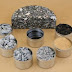Reviewed by ssorthiya58@gmail.com on 7/29/2020 01:34:00 PM Rating: 5